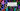# Reverse digits of a signed integer - Leet Code Solution

August 27, 2019## Problem Statement

Given a signed integer, reverse digits of an integer.

``````Example 1:
Input: 123
Output: 321

Example 2:
Input: -123
Output: -321

Example 3:
Input: 120
Output: 21``````

Return 0 for Integer overflow

## Algorithm

The algorithm should be simple. You fetch the last digit. For next round, you need to move this to next digit place, and add next last digit.

• Keep a flag indicating if the number is negative. Just to negate the result while returning And, make the number positive if it is negative
• You need to get last digit from number, and multiply last result by 10 to move it to next place.
``s = s*10 + remainder;``
• You need to take care of the integer overflow.

• We will do two calculations

• Multiply last remainder result by 10
• Add current remainder with above multiplication result
• `Overflow cases`: Note, max limit for 64-bit integer: 2^(64-1) - 1 = 2147483647

• `case 1`: Multiplication is itself overflowed: Operation we will do: s10, we can check if s10 > Integer.MAX_VALUE OR, s > Integer.MAX_VALUE/10

• `case 2`: if s*10 is equal to Integer.MAX_VALUE, Which means the number will be 214748364 multiply it with 10 will give: 21474836470 So, we need to check the remainder (which is to be added), if it is greater than 7, it is an overflow.

## Code

``````public class Q7_ReverseInteger {

public int reverse(int x) {
boolean neg = false;
if (x < 0) {
//negative number
neg = true;
x = -x;
}
int s = 0;
while (x > 0) {
int rem = x%10;

if (s > Integer.MAX_VALUE/10 || (s == Integer.MAX_VALUE/10 && rem > 7)) {
return 0;
}

s = s*10 + rem;

x = x/10;
}

if (neg) {
return -s;
}
return s;
}
}``````

## Complexity

It is equal to the number of digits of the number. `O(l)`